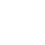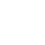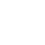Cms技巧

# ECSHOP模板自定义广告位的例子

```/*

* 调用广告位

*/

function index_ad_diy(\$p_id)

{

\$sql ='SELECT * FROM ' . \$GLOBALS['ecs']->table('ad') . ' where position_id ='.\$p_id.' ORDER BY ad_id desc limit 1';

\$res = \$GLOBALS['db']->getAll(\$sql);

\$arr = array();

foreach (\$res AS \$idx => \$row)

{

\$arr[\$idx]['id']          = \$row['ad_id'];

\$arr[\$idx]['title']       = \$row['ad_name'];

\$arr[\$idx]['imglink']       = \$row['ad_link'];

\$arr[\$idx]['imgcode']     = \$row['ad_code'];

}

return \$arr;

}```

`\$smarty->assign('ad_diy_left', index_ad_diy(29));`

```{foreach from=\$ad_diy_left  item=diyAd}
<a href="{\$diyAd.imglink}"><img src="data/afficheimg/{\$diyAd.imgcode}" alt="{\$diyAd.title}" title="{\$diyAd.title}" /></a>
{/foreach}```

TOPQQ客服18910140161